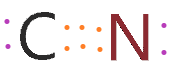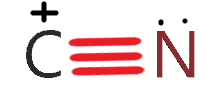# Cyanide (CN-) lewis structure, Molecular orbital diagram, bond order, formal charge, and its hybridization

Home  > Chemistry Article > CN- lewis structure and its molecular orbital diagramCyanide is a chemical compound with the chemical formula CN‾ ion. It is a member of the Cyano group. It is lethal for every living thing and smells like bitter almonds.

In this article, we will study the Cyanide (CN-) lewis structure, molecular orbital diagram(MO), its bond order, formal charges, and hybridization.

Cyanide can be a colorless gas in the form of hydrogen cyanide, sodium cyanide, potassium cyanide, etc. It is released as a decay product of many plants and it is one of the most poisonous chemicals in chemistry.

Some bacteria, fungi, algae, etc. also produced cyanide. It is used to manufacture paper, textiles, and plastics. Cyanide can be found in water, food, air, soil, etc. but it becomes highly dangerous when it is contacted with acid.

Properties of Cyanide(C≡N)

• Its molar mass is 26.018 g·mol−1
• Hydrogen cyanide is the conjugate acid of the CN group.
• It smells like bitter almonds.
• CN formal charge is -1.
• The cyanide anion is a ligand for many transition metals.
• The bond order of CN is 3.
• Cyanide ion formula is (C≡N)¯
Page Contents

## How to draw Cyanide (CN-) lewis structure?

CN lewis structure contains two atoms (carbon and nitrogen) connected with a triple bond. There is two lone pair present, one on nitrogen and the other on carbon in the CN lewis structure.

In this article, we will draw the lewis structure for CN, CN¯, CN+. Let’s start by taking CN¯(Cyanide ion).

## Steps to draw the lewis dot structure for CN¯ (Cyanide)

1. Count Valence electrons in CN¯

In the first step, count all valence electrons present in the CN molecule. As carbon belongs to the 14th group in the periodic table, so, it has 4 valence electrons and Nitrogen belongs to the 15th, so, it has 5 valence electrons.

⇒ Valence electron in carbon = 4

⇒ Valence electron in nitrogen = 5

∴ Total valence electron available for drawing the lewis structure of CN¯ = 4 + 5 + 1 = 10 valence electrons                 [∴ 1 is added because negative charge present on CN]In Cyanide lewis’s structure, we don’t need to find the least electronegativity or central atom as we find for other molecules. Because there are only two atoms present so, just place them adjacent to each other.2. Bonded together Carbon and Nitrogen with a single bond

In the second step, connect the carbon and nitrogen with a single bond.

###So, after bonding carbon and nitrogen, we used 2 electrons as one single bond contains two electrons. Now, we are left with 8 valence electrons.

3. Allocate remaining valence electron starting from outer atom first

In the third step, we need to locate the remaining electron on 2nd step structure. Start with the more electronegative atom or outer atom.

Clearly, nitrogen is more electronegative than carbon because electronegativity increase from left to right in the periodic table. Hence start with the nitrogen atom.

Nitrogen needs 6 more electrons to complete its octet rule as it already shares 2 electrons with a single bond. So, after putting 6 valence electrons on nitrogen, we are left with 2 electrons more.

Place these two electrons around the carbon atom.As you see in the above structure, nitrogen seems very happy as it gets 8 in its octet shell. But carbon seems disappointed as it is only getting 4 electrons. So, how would you make carbon happy by completing it octet?

Let’s see in the next step.

4. Fulfill all atoms octet by making a covalent bond

In this step, we will use a covalent bond to fulfill the octet of atoms if necessary. As in the 3rd step structure, Carbon not getting what it needs, so, simply convert the lone pair on nitrogen to a covalent bond.5. Locate charge on atoms

As we have to draw the lewis structure for CN¯. So, simply placed a negative charge on the above structure and complete it.### lewis structure for CN-

By following the same steps of the CN lewis structure, you can also make a lewis diagram for other cyanide ions.

### 1. lewis structure for CN∴ As there is only 9 valence electron present in CN molecule.

### 2. lewis structure for CN+∴ The total valence electron present in CN+ is 8

## Cyanide (CN-) hybridization and its formal charge

Hybridization of CN- is sp because carbon and nitrogen make the triple bond with each other. One bond is sigma and the other two are pi-bond.

Sigma bond means there is an end-to-end overlap of two orbitals.

Pi-bond means there is a side-to-side overlap of two p orbitals.

## What is the formal charge in CN- lewis’s structure and how to calculate it?

To calculate the formal charge in CN- lewis structure. Use this equation:

⇒ Formal charge = (Valence electrons – unbonded electrons – 1/2 bonded electrons)

⇒ First, calculate the formal charge on carbon.

∴ valence electron of carbon is 4

∴ non-bonding electrons are 2

∴ Bonding electrons is 6

= 4 – 2 – 6/2

= -1 is the formal charge on carbon

⇒ Now calculate the formal charge on Nitrogen

∴ valence electron of nitrogen is 5

∴ non-bonding electrons are 2

∴ Bonding electrons is 6

= 5 – 2 – 6/2

= 0 is the formal charge on nitrogen

So, the overall formal charge in CN¯ lewis structure is -1

## CN- molecular orbital diagram (MO) and its bond order

Molecular orbital theory explain the placement of electrons in a molecule. Generally, two types of bonding are formed by the combination of atomic orbitals. 1. Bonding orbital 2. Anti-bonding orbital.

Bonding orbital in which two atomic orbitals interact from the same phase and anti-bonding orbital in which two atomic interact from the different phase. Bonding orbital has less energy than anti-bonding orbital.

Electrons are fetched by lower energy orbitals first then they go to higher energy orbitals.

⇒ A homo-nuclear diatomic molecular orbital in which the same atoms combine together. example- N2, O2, B2, etc.

⇒ A hetero-nuclear diatomic molecular orbital in which different atoms combine together. example- CN, HF, NO, etc.

Clearly, Cyanide (CN) lies in a hetero-nuclear diatomic molecular orbital as it contains two different atoms. Also, using the Molecular orbital diagram of CN- we can also find its bond order which helps us to predict its bond length and stability as well.

## Procedure to draw the molecular orbital diagram of CN

1. Find the valence electron of each atom in the CN molecule. Clearly, carbon has 4 valence electrons and nitrogen has 5.

2. Find if the molecule homo-nuclear diatomic molecular orbital or hetero-nuclear diatomic molecular orbital. Clearly, CN is hetero orbital.

3. In the third step, fill the molecular orbitals using the energy and bonding properties of the overlapping atomic orbitals.

4. Draw the MO diagram of CN and fill it with electrons. After that, with the help of the CN Molecular orbital diagram, you can easily predict its properties like bond order, bond length, para-magnetic, or diamagnetic, etc.### What is the bond order of CN–?

To find the bond order of CN. First, know its formula.

∴ Bond order = (Nb – N)/2

⇒ Nb = Number of bonding electrons

⇒ Na = Number of anti-bonding electrons

⇒ The total number of electrons in CN is 14 of which 10 are anti-bonding and 4 bonding electrons.

⇒ And electronic configuration of CN is (σ1s)2(σ*1s)2(σ2s)2(σ*2s)2(π2px)2(π2py)2.

∴ Simply put these in the formula to find the bond order of CN.

= (10 – 4)/2

= 3 is the bond order of CN

The bond order of CN+ is 2 because it contains 12 valence electrons.

## FAQ

### How many lone pairs are in the CN, CN-, CN+ lewis structure?

According to the lewis structure of Cyanide-

⇒ CN = It has one lone pair on the nitrogen atom

⇒ CN+ = It also has one pair on the nitrogen atom

⇒ CN- = It has two lone pairs, one along with nitrogen and one along with carbon.

### Is CN polar or nonpolar molecule according to its lewis structure?

CN is a polar molecule because large electronegativity difference present in carbon and nitrogen. Also, nitrogen is more electronegative than carbon so it attracts more electrons.

Nitrogen has a partial negative charge and unequal charge distribution as we saw in the CN lewis structure. All these factors lead to Cyanide (CN) become a polar molecule.

### Why cyanide has a negative charge?

As we see in the cyanide ion lewis dot structure, a negative charge and a lone pair are present on the carbon atom. Combining lone pair and negative charge makes carbon end nucleophile. Thus, cyanide has a negative charge.

## Summary

So, in this article, we have learned about How to draw CN lewis structure, its molecular orbital diagram(MO), formal charges, hybridization, and bond order. Here is a quick review of this article.

• Hybridization of CN is Sp.
• The bond order of CN- is 3.
• CN formal charge is -1 according to its lewis structure.
• The total valence electron available for CN Lewis structure is 10.
• Total Lone pair on CN- the molecule is 2.
• CN is polar molecular.
##### Subscribe to Blog via Email

Join 2 other subscribers

Share it...